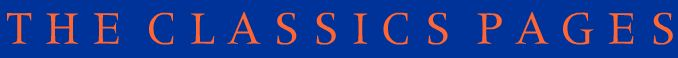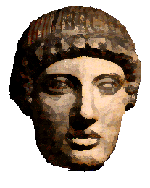# The Maths-Classics project

## Assignment 2

### The Greeks

Much of mathematics was discovered by the Greeks, and so many of the words have a Greek derivation. Below are the Greek numbers from 1 to 13 and in brackets are the forms of these words that are used in mathematical terms.

1. heis, mia, hen

2. dyo

3. treis ( tri- )

4. tettares ( tetra- )

5. pente ( penta- )6. hex ( hexa- )

7. hepta

8. okto ( octa- )

9. ennea

10. deka ( deca- )

11. hendeka

12. dodeka (dodeca-)

13. triskaideka

Use your dictionary to see if you can find the numbers which are associated with the following Greek words:

eikosi ( icosa- )

hekaton ( hecto- )

chilioi ( kilo- )

There are also some useful Greek PREFIXES (i.e. words which are put at the start of a longer word).

poly - many

dia - through

panto - all

iso - equal

geo - earth

arithmo - number

hemi - half

mono - ?

micro - ?

mega - ?

nano - dwarf

There are also some useful Greek SUFFIXES (i.e. words which are put at the end of a longer word)

 -morph shape -phobia fear -graph or -gram something written or drawn -gon corner -hedron seat or bottom, used in regular solids -meter (UK -metre) measure, measuring -logy study of

Draw and label clearly the following:

pentagon, decagon, octagon, dodecagon, polygon ( other than the previous ones )

Write down the words for:

1. study of the earth

2. writing or drawing about the earth

3. a solid with four sides

4. fear of numbers

5. fear of number 13

6. something with many shapes

Try to work out the literal meaning of the following words and see how this has been altered to give the meaning in your English dictionary:

pentagram, pantograph, trigonometry, diameter, icosahedron, dodecahedron

See if you can make up words for the following:

1. a monster with 13 shapes

2. fear of everything

3. study of the shape of the earth ( check if this word actually exists )

Can you make up any words of your own from the Greek numbers, prefixes and suffixes? What would they mean?

### Metric System:

In assignment 1, Latin words with a unit ( e.g centimetre ) meant smaller units. A Greek prefix more often means a larger unit (what's a hect-are?) - but in the SI system (what is this?) some Greek terms are for VERY VERY SMALL units. Arrange these on order of size (smallest first) and say whether they are Greek or Latin. Find out exactly what they mean mathematically. They are usually written as (for example) 10-6. Which -metre is this? And which Greek letter is used as a symbol for it?

1. milli-
2. micro-
3. nano-
4. mega-
5. kilo-

Find some examples of units using these prefixes (eg megawatt, nanometre). Make up some of your own - using these or other prefixes - then check whether thay actually exist.

We still use Greek letters in Mathematics today. Can you write out the complete Greek alphabet? There are two symbols for each letter - an ordinary one (lower case or minuscule) and a capital (upper case).

The names and nearest Engish equivalents are :

 alpha a beta b gamma g delta d epsilon e zeta z eta e theta th iota i kappa k lambda l mu m nu n xi x omicron o pi p rho r sigma s tau t upsilon u phi ph chi ch psi ps omega o

Here's a site where you can learn and practise the Greek alphabet.